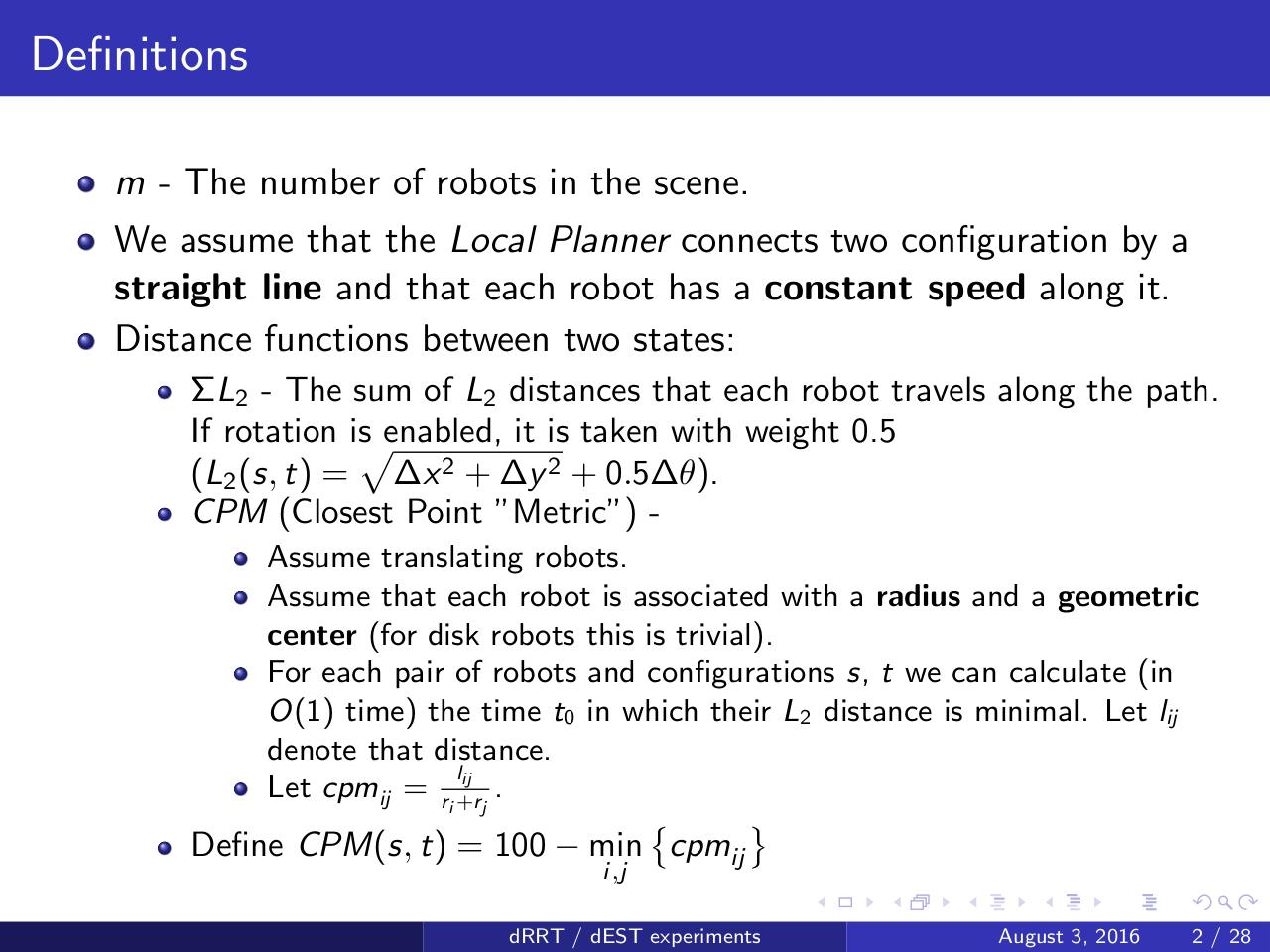# dRRT ex.pdfPage 1 23428

#### Text preview

Definitions
m - The number of robots in the scene.
We assume that the Local Planner connects two configuration by a
straight line and that each robot has a constant speed along it.
Distance functions between two states:
ΣL2 - The sum of L2 distances that each robot travels along the path.
If rotation ispenabled, it is taken with weight 0.5
(L2 (s, t) = ∆x 2 + ∆y 2 + 0.5∆θ).
CPM (Closest Point ”Metric”) Assume translating robots.
Assume that each robot is associated with a radius and a geometric
center (for disk robots this is trivial).
For each pair of robots and configurations s, t we can calculate (in
O(1) time) the time t0 in which their L2 distance is minimal. Let lij
denote that distance.
lij
Let cpmij = ri +r
.
j



Define CPM(s, t) = 100 − min cpmij
i,j

dRRT / dEST experiments

August 3, 2016

2 / 28